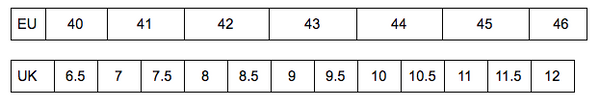# Myrqvist Goodyear Welted - SizechartNot sure what size to choose?

If you know that you, for example, have size EU 42, but slightly lean towards a bigger size (EU 42.5 / EU 42 ⅔ ) we recommend that you choose the bigger size in our shoes. In this case that would be size 8.5.

Below you’ll find the inner length of our shoes in each different size.

• UK 6.5 = 275 millimeters
• UK 7 = 279 millimeters
• UK 7.5 = 283 millimeters
• UK 8 = 287 millimeters
• UK 8.5 = 291 millimeters
• UK 9 = 295 millimeters
• UK 9.5 = 299 millimeters
• UK 10 = 303 millimeters
• UK 10.5 = 307 millimeters
• UK 11 = 311 millimeters
• UK 11.5 = 315 millimeters
• UK 12 = 319 millimeters